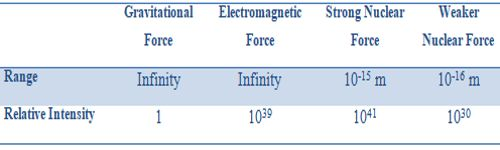Physics

# Comparison of the Intensities of Fundamental ForcesComparison of the Intensities of Fundamental Forces:

Among the four fundamental forces, strongest force is the strong nuclear force and weakest one is the gravitational force. The range of both strong and weak nuclear forces are short, whereas the range of gravitational and electromagnets forces are almost infinity.

If the relative strength of the four fundamental forces is compared, it is seen that strong nuclear force is the strongest one and the weakest force is the gravitational force. The range of both strong and weak nuclear forces is that range. These forces are not active outside the nucleus. On die other hands, a range of the gravitational and electromagnetic forces is almost infinity.

Look at the following table to know the relative strength of the four fundamental forces.

Table: Comparison of the fundamental forces –Four types of fundamental forces are there in nature. They are –

• Gravitational force; weakest force; but an infinite range.
• Electromagnetic force; stronger, with infinite range.
• Strong nuclear force, strongest; but short range.
• Weak nuclear force; next weakest; but short range.